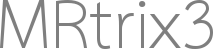# Wrong FOD orientations

hello,

i am working with some slightly problematic data that is stored solely as the SH coefficients in 4D matrix in matlab format (sh_mat = 120x120x100x15 matrix) and i am trying to convert it to .mif using the write_mrtrix command. the data has a transform associated with it

``````M =	-2     0     0   122
0    -2     0   122
0     0    -2   102
0     0     0     1

fod.data = sh_mat;
fod.transform = M;
``````

and `write_mrtrix(fod, 'outfod.mif')` gives the wrong orientation in the corpus callosum (obv they’re all wrong but it’s noticeable in the CC). you can also see it in the projections to the cortex.

I’ve tried a bunch of different ways to flip the orientations but nothing’s worked, any suggestions on this would be great.

thanks!

Hi Diliana,

It looks like your gradient table and DWI image do not match so you’d need to look into your acquisition and processing pipeline. `dwigradcheck` might be handy.

Unrelated but the determinant of your transformation matrix `M` is negative, meaning you’re flipping your image.

Cheer,
Max

I think the issue might be related to those negative entries on the diagonal of your transform – as @maxpietsch pointed out. There’s a good chance that’ll flip all the axes. Have you tried making all of those positive?

Otherwise, where do these data come from? If they’re using different conventions from MRtrix, we’d need to take a close look at what these are. There’s a lot of ways this information can be represented - even if it is all valid SH coefficients…

Hi Donald and MaxSo making it positive does not help:

so the data are an average fod. SH coeffs of individual subjects are estimated in native space using in-house code for RSI (this is the original paper) then subjects are registered together using a tensor registration and the native space SH are transformed to the common space and average, and the average is what i’ve got here. the registration/transformation/averaging is done by someone else so i don’t know the details but will find out (all this processing has been done before me/by other people)

but i see the same problem when i try to convert subjects native space SH to .mif, so before any transformation of the data which makes me think it’s more about how the data are stored. also i did a quick check to see what i get using dwi2fod, expecting if there was a problem with the gradients that the fod orientations would again be messed up, but that looks correctly orientated.

also i wanted to say this but it only would let me post one image:
i probably should have mentioned this earlier but when i get the data if i just do write_mrtrix the on the sh_mat as is i get:

but re-ordering the SH indexing to 1 6 5 4 3 2 15 14 13 12 11 10 9 8 7 gives me what i showed in the first post.

Ok, then I’m assuming the issue is indeed that our SH storage conventions differ from the package you’re using – it certainly seems like the order is different, since you’ve already dealt with that. The remaining issues might relate to inverting some of coefficients or something like that – for instance, there’s a (-1)^m term (the Condon–Shortley phase) that features in some conventions but not others. The way we combine the complex SH terms to form a real basis (sometimes called the tesseral spherical harmonics) might also assume different conventions. Lots of ways things can end up defined differently… Maybe if you can find the exact definitions assumed in their code, we can figure out the mapping between the two?

success! i think… looking at the original maltab code

``````for l = 0:2:order
p = legendre(l,cos(Theta)');
for m = -l:l
j = (l^2 + l + 2)/2 + m;
if m<0
c1 = factorial(l-m)/factorial(l+m);
c2 = (2*l+1)/(4*pi);
c3 = (-1)^(abs(m))*(factorial(l-abs(m))/factorial(l+abs(m)));
Ylm = sqrt(c1*c2)*c3*p(abs(m)+1,:)'.*exp(i*m*Phi);
Y(:,j) = sqrt(2)*real(Ylm);
elseif m>0
c1 = factorial(l-m)/factorial(l+m);
c2 = (2*l+1)/(4*pi);
Ylm = sqrt(c1*c2)*p(m+1,:)'.*exp(i*m*Phi);
Y(:,j) = sqrt(2)*imag(Ylm);
else
c1 = factorial(l-m)/factorial(l+m);
c2 = (2*l+1)/(4*pi);
Ylm = sqrt(c1*c2)*p(m+1,:)'.*exp(i*m*Phi);
Y(:,j) = Ylm;
end
end
end
``````

the `m<0` term has a `(-1)^(abs(m))` so multiplying the negative degree coefficients by `(-1)^(abs(m))` gives (apparently) correct orientations.

but to be honest i don’t really understand the `c3` term for `m<0`.

Good to hear!

Me neither… But there’s a lot of redundancy in this code. I reckon this would do the same job:

``````for l = 0:2:order
p = legendre(l,cos(Theta)');
for m = -l:l
j = (l^2 + l + 2)/2 + m;
c1 = factorial(l-abs(m))/factorial(l+abs(m));
c2 = (2*l+1)/(4*pi);
Ylm = sqrt(c1*c2)*p(abs(m)+1,:)'.*exp(i*m*Phi);
if m<0
Y(:,j) = sqrt(2)*(-1)^(abs(m))*real(Ylm);
elseif m>0
Y(:,j) = sqrt(2)*imag(Ylm);
else
Y(:,j) = Ylm;
end
end
end
``````

and there’s a good chance that would then match our convention, apart from that `(-1)^abs(m)` term, and the need to invert m – but to really verify this would require writing a bit of test code…

thanks donald! hope all is well back in london!

1 Like